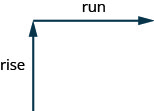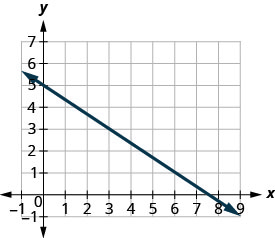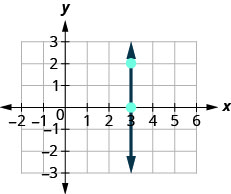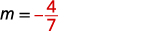# 3.3: 直线的斜率

•• OpenStax
• OpenStax
$$\newcommand{\vecs}{\overset { \rightharpoonup} {\mathbf{#1}} }$$ $$\newcommand{\vecd}{\overset{-\!-\!\rightharpoonup}{\vphantom{a}\smash {#1}}}$$$$\newcommand{\id}{\mathrm{id}}$$ $$\newcommand{\Span}{\mathrm{span}}$$ $$\newcommand{\kernel}{\mathrm{null}\,}$$ $$\newcommand{\range}{\mathrm{range}\,}$$ $$\newcommand{\RealPart}{\mathrm{Re}}$$ $$\newcommand{\ImaginaryPart}{\mathrm{Im}}$$ $$\newcommand{\Argument}{\mathrm{Arg}}$$ $$\newcommand{\norm}{\| #1 \|}$$ $$\newcommand{\inner}{\langle #1, #2 \rangle}$$ $$\newcommand{\Span}{\mathrm{span}}$$ $$\newcommand{\id}{\mathrm{id}}$$ $$\newcommand{\Span}{\mathrm{span}}$$ $$\newcommand{\kernel}{\mathrm{null}\,}$$ $$\newcommand{\range}{\mathrm{range}\,}$$ $$\newcommand{\RealPart}{\mathrm{Re}}$$ $$\newcommand{\ImaginaryPart}{\mathrm{Im}}$$ $$\newcommand{\Argument}{\mathrm{Arg}}$$ $$\newcommand{\norm}{\| #1 \|}$$ $$\newcommand{\inner}{\langle #1, #2 \rangle}$$ $$\newcommand{\Span}{\mathrm{span}}$$$$\newcommand{\AA}{\unicode[.8,0]{x212B}}$$

##### 学习目标

• 找出直线的斜率
• 给定一个点和斜率绘制一条直线
• 使用斜率和截距绘制直线图
• 选择最方便的方法来绘制线条
• 绘制和解释坡度截距的应用
• 使用斜率识别平行线和垂直线

1. 简化：$$\frac{(1–4)}{(8−2)}$$
如果你错过了这个问题，请查看 [链接]
2. 除以:$$\frac{0}{4}$$,$$\frac{4}{0}$$
如果你错过了这个问题，请查看 [链接]
3. 简化：$$\frac{15}{-3}$$$$\frac{-15}{3}$$$$\frac{-15}{-3}$$
如果你错过了这个问题，请查看 [链接]

## 找出直线的斜率图$$\PageIndex{1}$$。

##### 使用以下方法从直线图中找出直线的斜率$$m=\frac{\text{rise}}{\text{run}}$$
1. 在坐标为整数的直线上找到两个点。
2. 从一个点开始，画一个直角三角形，从第一个点到第二个点。
3. 计算三角形腿上的上升和跑步。
4. 取上升比率得出斜率:$$m=\frac{\text{rise}}{\text{run}}$$.
##### 示例$$\PageIndex{1}$$在图表上找到 坐标为整数的两个点。 $$(0,5)$$和$$(3,3)$$ 从开始$$(0,5)$$，绘制一个直角三角形 $$(3,3)$$，如下图所示。算上涨幅度——既然下跌，就是负数。 上升是$$−2$$。 计算跑步次数。 跑步是 3。 使用斜率公式。 $$m=\frac{\text{rise}}{\text{run}}$$ 替换上升和运行的值。 $$m=−23$$ 简化。 $$m=−23$$ 直线的斜率为$$−23$$。 因此，当 x 增加 3 个单位时，y 会减少 2 个单位。
##### 示例$$\PageIndex{2}$$$$-\frac{4}{3}$$

##### 示例$$\PageIndex{3}$$$$-\frac{3}{5}$$$$\begin{array} {ll} {\text{What is the rise?}} &{\text{The rise is }0.} \\ {\text{What is the run?}} &{\text{The run is }3.} \\ {\text{What is the slope?}} &{m=\frac{\text{rise}}{\text{run}}} \\ {} &{m=\frac{0}{3}} \\ {} &{m=0} \\{}&{\text{The slope of the horizontal line } y=4 \text{ is }0.} \\ \end{array} \nonumber$$$$\begin{array} {ll} {\text{What is the rise?}} &{\text{The rise is }0.} \\ {\text{What is the run?}} &{\text{The run is }3.} \\ {\text{What is the slope?}} &{m=\frac{\text{rise}}{\text{run}}} \\ {} &{m=\frac{2}{0}} \\ \end{array} \nonumber$$

##### 示例$$\PageIndex{4}$$

$$x=8$$

$$y=−5$$

0

##### 线条斜率快速指南$$\begin{array} {ll} {(x_1, y_1)} &{\text{read “} x \text{ sub } 1, \space y \text{ sub } 1 \text{”}} \\ {(x_2, y_2)} &{\text{read “} x \text{ sub } 2, \space y \text{ sub } 2 \text{”}} \\ \end{array} \nonumber$$$$\begin{array} {ll} {\text{Since we have two points, we will use subscript notation.}} &{ \begin{pmatrix} x_1, & y_1 \\ 2 & 3 \end{pmatrix} \begin{pmatrix} x_2, & y_2 \\ 6 & 6 \end{pmatrix}} \\ {} &{m=\frac{\text{rise}}{\text{run}}} \\ {\text{On the graph, we counted the rise of 3 and the run of 5.}} &{m=\frac{3}{5}} \\ {\text{Notice that the rise of 3 can be found by subtracting the}} &{} \\ {y\text{-coordinates, 6 and 3, and the run of 5 can be found by}} &{} \\ {\text{subtracting the x-coordinates 7 and 2.}} &{} \\ {\text{We rewrite the rise and run by putting in the coordinates.}} &{m=\frac{6-3}{7-2}} \\ {} &{} \\ {\text{But 6 is } y_2 \text{, the y-coordinate of the second point and 3 is }y_1 \text{, the y-coordinate}} &{} \\ {\text{of the first point. So we can rewrite the slope using subscript notation.}} &{m=\frac{y_2-y_1}{7-2}} \\ {\text{Also 7 is the x-coordinate of the second point and 2 is the x-coordinate}} &{} \\ {\text{of the first point. So again we rewrite the slope using subscript notation.}} &{m=\frac{y_2-y_1}{x_2-x_1}} \\ \end{array} \nonumber$$

##### 两点之间的直线的斜率

$$m=\frac{y_2−y_1}{x_2−x_1}$$

$y\text{ of the second point minus }y\text{ of the first point} \nonumber$$\text{over} \nonumber$$x\text{ of the second point minus }x\text{ of the first point} \nonumber$

##### 示例$$\PageIndex{7}$$

$$\begin{array} {ll} {\text{We’ll call (−2,−3) point #1and (−7,4) point #2.}} &{ \begin{pmatrix} x_1, & y_1 \\ -2 & -3 \end{pmatrix} \begin{pmatrix} x_2, & y_2 \\ -7 & 4 \end{pmatrix}} \\ {\text{Use the slope formula.}} &{m=\frac{y_2-y_1}{x_2-x_1}} \\ {\text{Substitute the values.}} &{} \\ {\text{y of the second point minus y of the first point}} &{} \\ {\text{x of the second point minus x of the first point}} &{m=\frac{4-(-3)}{-7-(-2)}} \\{\text{Simplify}}&{m=\frac{7}{-5}} \\ {} &{m=\frac{-7}{5}} \\ \end{array} \nonumber$$$m=\frac{\text{rise}}{\text{run}} \nonumber$$m=\frac{7}{−5} \nonumber$$m=\frac{−7}{5} \nonumber$

$$-1$$

10

## 给定一个点和斜率绘制一条直线

##### 示例$$\PageIndex{10}$$: How to graph a Line Given a Point and the Slope##### 示例$$\PageIndex{11}$$##### 示例$$\PageIndex{12}$$##### 给定一个点和斜率绘制一条直线。
1. 绘制给定点。
2. 使用斜率公式$$m=\frac{\text{rise}}{\text{run}}$$来确定上升和游程。
3. 从给定点开始，计算上涨幅度，然后跑到第二个点。
4. 用直线连接各点。

## 使用斜率和截距绘制直线图

。 让我们看一下方程$$y=12x+3$$的图形，找出它的斜率和 y 截距。图$$\PageIndex{2}$$

$m=\frac{\text{rise}}{\text{run}} \nonumber$$m=\frac{1}{2} \nonumber$

y 截距为$$(0,3)$$##### 示例$$\PageIndex{13}$$

$$y=−\frac{4}{7}x−2$$$$x+3y=9$$

ⓐ 我们将方程与方程的坡度截距形式进行比较。

 写下直线方程的斜率截距形式。写下直线的方程式。确定斜率。识别 y 截距。ⓑ 当直线的方程没有以斜率截距形式给出时，我们的第一步将是求解 y 的方程。

 求解 y。 x+3y=9x+3y=9 从两边减去 x。将两边除以 3。简化。写下直线方程的斜率截距形式。写下直线的方程式。确定斜率。识别 y 截距。##### 示例$$\PageIndex{14}$$

$$y=\frac{2}{5}x−1$$$$x+4y=8$$

$$m=\frac{2}{5}$$;$$(0,−1)$$
$$m=−\frac{1}{4}$$;$$(0,2)$$

##### 示例$$\PageIndex{15}$$

$$y=−\frac{4}{3} x+1$$$$3x+2y=12$$

$$m=−\frac{4}{3}$$;$$(0,1)$$
$$m=−\frac{3}{2}$$;$$(0,6)$$

##### 示例$$\PageIndex{16}$$

 $$y=mx+b$$ 方程采用斜率截距形式。 $$y=−x+4$$ 确定斜率和 y 截距。 $$m=−1$$ y-截距为$$(0,4)$$ 绘制 y 截距。 参见图表。 确定跑步过程中的涨幅。 $$m=−11$$ 计算出上升幅度，然后跑到第二个点。 上升$$-1$$，跑$$1$$##### 示例$$\PageIndex{17}$$

$$y=−x−3$$使用方程的斜率和 y 截距绘制方程的直线。##### 示例$$\PageIndex{18}$$

$$y=−x−1$$使用方程的斜率和 y 截距绘制方程的直线。## 选择最方便的方法来绘制线条

$\begin{array} {lll} {} &{\textbf{Equation}} &{\textbf{Method}} \\ {\text{#1}} &{x=2} &{\text{Vertical line}} \\ {\text{#2}} &{y=−1} &{\text{Horizontal line}} \\ {\text{#3}} &{−x+2y=6} &{\text{Intercepts}} \\ {\text{#4}} &{4x−3y=12} &{\text{Intercepts}} \\ {\text{#5}} &{y=−x+4} &{\text{Slope–intercept}} \\ \end{array} \nonumber$

##### 选择最便捷的绘制线条方法的策略

• 如果它只有一个变量，则它是一条垂直线或水平线。
• $$x=a$$是一条在 a 处穿过 x 轴的垂直线。
• $$y=b$$是一条在 b 处穿过 y 轴的水平线。
• 如果 y 在方程的一侧是孤立的，则以表格形式$$y=mx+b$$使用斜率和 y 截距绘制图形。
• 确定斜率和 y 截距，然后绘制图形。
• 如果方程的形式为$$Ax+By=C$$，则找出截距。
• 找到 xy 截距，第三个点，然后绘制图表。
##### 示例$$\PageIndex{19}$$

$$y=5$$$$4x−5y=20$$$$x=−3$$$$y=−\frac{5}{9}x+8$$

$$y=5$$

$$4x−5y=20$$

$$x=−3$$

$$y=−\frac{5}{9}x+8$$

##### 示例$$\PageIndex{20}$$

$$3x+2y=12$$$$y=4$$$$y=\frac{1}{5}x−4$$$$x=−7$$

ⓐ 拦截 ⓑ 水平线 ⓒ slope-intercept ⓓ 垂直线

##### 示例$$\PageIndex{21}$$

$$x=6$$$$y=−\frac{3}{4}x+1$$$$y=−8$$$$4x−3y=−1$$

ⓐ 垂直线 ⓑ 斜率截距 ⓒ 水平线
ⓓ 截距

## 绘制和解释斜率截距的应用

##### 示例$$\PageIndex{22}$$

$$F=\frac{9}{5}C+32$$该方程用于将摄氏度上的温度 C 转换为华氏度上的温度 F

ⓐ 找到摄氏温度为 0 的华氏温度。

ⓑ 找出摄氏温度 20 摄氏度的华氏温度。

ⓒ 解释方程的斜率和 F 截距。

ⓓ 绘制方程图。

$$\begin{array} {ll} {\text{Find the Fahrenheit temperature for a Celsius temperature of 0.}} &{F=\frac{9}{5}C+32} \\ {\text{Find F when C=0.}} &{F=\frac{9}{5}(0)+32} \\ {\text{Simplify.}} &{F=32} \\ \end{array} \nonumber$$

$$\begin{array} {ll} {\text{Find the Fahrenheit temperature for a Celsius temperature of 20.}} &{F=\frac{9}{5}C+32} \\ {\text{Find F when C=20.}} &{F=\frac{9}{5}(20)+32} \\ {\text{Simplify.}} &{F=36+32} \\ {\text{Simplify.}} &{F=68} \\ \end{array} \nonumber$$F 截距意味着当温度达到摄氏度时，它$$32°$$在华氏度上。$$0°$$
ⓓ 绘制方程图。##### 示例$$\PageIndex{23}$$

ⓐ 估算穿女鞋尺码 0 的孩子的身高。

ⓑ 估算穿着 8 号鞋子的女士的身高。

ⓒ 解释方程的斜率和 h 截距。

ⓓ 绘制方程图。

ⓐ 50 英寸
ⓑ 66 英寸
ⓒ 斜率 2 表示当鞋码 s 增加 1 时，高度 h 增加 2 英寸。 h 截距表示当鞋码为 0 时，高度为 50 英寸。##### 示例$$\PageIndex{24}$$

ⓐ 估计没有啁时的温度。

ⓑ 当一分钟内的啁数为 100 时，估计温度。

ⓒ 解释方程的斜率和 T 截距。

ⓓ 绘制方程图。

ⓐ 40 度
ⓑ 65 度
ⓒ 斜率表示当啁数 n 增加 4 时，温度华氏度 (F) 增加 1 度。$$\frac{1}{4}$$ T 截距表示当啁数为 0 时，温度为 40°。##### 示例$$\PageIndex{25}$$

ⓐ 查找 Sam 行驶 0 英里时一周的费用。

ⓑ 找出他行驶 250 英里一周的费用。

ⓒ 解释方程的斜率和 C 截距。

ⓓ 绘制方程图。

$$\begin{array} {ll} {\text{Find Sam’s cost for a week when he drives 0 miles.}} &{C=0.5m+60} \\ {\text{Find C when m=0.}} &{C=0.5(0)+60} \\ {\text{Simplify.}} &{C=60} \\ {} &{\text{Sam’s costs are }\text{60 when he drives 0 miles.}} \\ \end{array} \nonumber$$

$$\begin{array} {ll} {\text{Find Sam’s cost for a week when he drives 250 miles.}} &{C=0.5m+60} \\ {\text{Find C when m=250.}} &{C=0.5(250)+60} \\ {\text{Simplify.}} &{C=185} \\ {} &{\text{Sam’s costs are }\text{185 when he drives 250 miles.}} \\ \end{array} \nonumber$$
ⓒ 解释方程的斜率和 C 截距。C-intercept 表示当行驶里程数为 0 时，每周成本为 60 美元。
ⓓ 绘制方程图。

$$\begin{array} {ll} {} &{m=0.5} \\ {\text{Rewrite as a fraction.}} &{m=\frac{0.5}{1}} \\ {\text{Multiply numerator and}} &{} \\ {\text{denominator by 100}} &{m=\frac{0.5(100)}{1(100)}} \\ {\text{Simplify.}} &{m=\frac{50}{100}} \\ \end{array} \nonumber$$##### 示例$$\PageIndex{26}$$

ⓐ 查看 Stella 不卖披萨一周的费用。

ⓑ 找出她卖出 15 个披萨一周的费用。

ⓒ 解释方程的斜率和 C 截距。

ⓓ 绘制方程图。

ⓐ $25 ⓑ$85
ⓒ 斜率为 4，表示当售出的披萨数量 p 增加 1 时，每周成本 C 增加 4 美元。 C-intercept 表示当售出的披萨数量为 0 时，每周成本为 25 美元。##### 示例$$\PageIndex{27}$$

ⓐ 查看 Loreen 没有写邀请函时一周的费用。

ⓑ 找出她写了 75 份邀请函时一周的费用。

ⓒ 解释方程的斜率和 C 截距。

ⓓ 绘制方程图。

ⓐ $35 ⓑ$170
ⓒ 斜率表示当邀请数量 n 增加 1$$1.80$$ 时，每周成本 C 会增加。$$1.8$$
C-intercept 表示当邀请数量为 0 时，每周费用为 35 美元。## 使用斜率识别平行线和垂直线图$$\PageIndex{3}$$图$$\PageIndex{4}$$
##### 平行线

• 平行线具有相同的斜率和不同的 y 截距。
• 如果 m1m1 和 m2m2 是两条平行线的斜率，那么 m1=m2.m1=m2。
• 平行垂直线有不同的 x 截距

##### 示例$$\PageIndex{28}$$

$$3x−2y=6$$$$y=\frac{3}{2}x+1$$$$y=2x−3$$$$−6x+3y=−9$$

$$\begin{array} {llll} {} &{3x−2y=6} &{\text{and}} &{y=\frac{3}{2}x+1} \\ {} &{−2y=−3x+6} &{} &{} \\ {\text{Solve the first equation for y.}} &{\frac{-2y}{-2}=\frac{-3x+6}{-2}} &{} &{} \\ {\text{The equation is now in slope–intercept form.}} &{y=\frac{3}{2}x−3} &{} &{} \\ {\text{The equation of the second line is already}} &{} &{} &{} \\ {\text{in slope–intercept form.}} &{} &{} &{y=\frac{3}{2}x+1} \\ {} &{} &{} &{} \\ {} &{y=\frac{3}{2}x−3} &{} &{y=\frac{3}{2}x+1} \\ {Identify the slope andy-intercept of both lines.} &{y=mx+b} &{} &{y=mx+b} \\ {} &{m=\frac{3}{2}} &{} &{y=\frac{3}{2}} \\ {} &{\text{y-intercept is }(0,−3)} &{} &{\text{y-intercept is }(0,1)} \\ \end{array} \nonumber$$

$$\begin{array} {llll} {} &{y=2x−3} &{\text{and}} &{−6x+3y=−9} \\ {\text{The first equation is already in slope–intercept form.}} &{y=2x−3} &{} &{} \\ {} &{} &{} &{−6x+3y=−9} \\ {} &{} &{} &{3y=6x−9} \\ {\text{Solve the second equation for y.}} &{} &{} &{\frac{3y}{3}=\frac{6x−9}{3}} \\ {} &{} &{} &{y=2x−3} \\ {\text{The second equation is now in slope–intercept form.}} &{} &{} &{y=2x−3} \\ {} &{} &{} &{} \\ {} &{y=2x−3} &{} &{y=2x−3} \\ {\text{Identify the slope andy-intercept of both lines.}} &{y=mx+b} &{} &{y=mx+b} \\ {} &{m=2} &{} &{m=2} \\ {} &{\text{y-intercept is }(0,−3)} &{} &{\text{y-intercept is }(0,-3)} \\ \end{array} \nonumber$$

##### 示例$$\PageIndex{29}$$

$$2x+5y=5$$$$y=−\frac{2}{5}x−4$$$$y=−\frac{1}{2}x−1$$$$x+2y=−2$$

ⓐ 平行 ⓑ 不平行；同一条线

##### 示例$$\PageIndex{30}$$

$$4x−3y=6$$$$y=\frac{4}{3}x−1$$$$y=\frac{3}{4}x−3$$$$3x−4y=12$$

ⓐ 平行 ⓑ 不平行；同一条线

##### 示例$$\PageIndex{31}$$

$$y=−4$$$$y=3$$$$x=−2$$$$x=−5$$

$$y=−4$$$$y=3$$

$$x=−2$$$$x=−5$$

##### 示例$$\PageIndex{32}$$

$$y=8$$$$y=−6$$$$x=1$$$$x=−5$$

ⓐ 平行 ⓑ 平行

##### 示例$$\PageIndex{33}$$

$$y=1$$$$y=−5$$$$x=8$$$$x=−6$$

ⓐ 平行 ⓑ 平行图$$\PageIndex{5}$$

$\begin{array} {l} {m_1·m_2} \\ {14(−4)} \\ {−1} \\ \end{array} \nonumber$

##### 垂直线

• 如果$$m_1$$$$m_2$$是两条垂直线的斜率，则：
• 它们的斜率是彼此的负倒数，$$m_1=−\frac{1}{m_2}$$
• 他们斜率的乘积是$$−1$$$$m_1·m_2=−1$$
• 垂直线和水平线始终相互垂直

##### 示例$$\PageIndex{34}$$

$$y=−5x−4$$$$x−5y=5$$$$7x+2y=3$$$$2x+7y=5$$

##### 示例$$\PageIndex{3}$$

$$y=−3x+2$$$$x−3y=4$$$$5x+4y=1$$$$4x+5y=3$$

ⓐ 垂直 ⓑ 不垂直

##### 示例$$\PageIndex{3}$$

$$y=2x−5$$$$x+2y=−6$$$$2x−9y=3$$$$9x−2y=1$$

ⓐ 垂直 ⓑ 不垂直

## 关键概念

• 直线的斜率
• 直线的斜率为$$m=\frac{\text{rise}}{\text{run}}$$
• 上升衡量垂直变化，运行衡量水平变化。

• 如何使用图表中找到直线的斜率$$m=\frac{\text{rise}}{\text{run}}$$
1. 在坐标为整数的直线上找到两个点。
2. 从一个点开始，画一个直角三角形，从第一个点到第二个点。
3. 计算三角形腿上的上升和跑步。
4. 取上升比率得出斜率:$$m=\frac{\text{rise}}{\text{run}}$$.
• 两点之间的直线的斜率。
• 两点$$(x_1,y_1)$$和之间的直线的斜率$$(x_2,y_2)$$为：

$m=\frac{y_2−y_1}{x_2−x_1} \nonumber$

• 如何在给定点和斜率的情况下绘制直线。
1. 绘制给定点。
2. 使用斜率公式$$m=\frac{\text{rise}}{\text{run}}$$来确定上升和游程。
3. 从给定点开始，计算上涨幅度，然后跑到第二个点。
4. 用直线连接各点。
• 直线方程的斜率截距形式
• 斜率为 my 截距的直线方程的斜率截距形式$$(0,b)$$$$y=mx+b$$
•• 平行线
• 平行线是同一平面中不相交的线。
平行线具有相同的斜率和不同的 y 截距。
如果$$m_1$$$$m_2$$是两条平行线的斜率，那么$$m_1=m_2$$
平行垂直线有不同的 x 截距。
• 垂直线
• 垂直线是同一平面上形成直角的直线。
• 如果$$m_1$$$$m_2$$是两条垂直线的斜率，那么：
它们的斜率是彼此的负倒数，$$m_1=−\frac{1}{m_2}$$
他们斜率的乘积是$$−1$$$$m_1·m_2=−1$$
• 垂直线和水平线始终相互垂直。